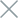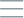• Home
• Tags
• Series
•# Multiple-Item Search Algorithm in C++ - Explanation, Example, and Code

The multiple-item search algorithm is used to find all occurrences of a specific element in a list. Unlike single-item search algorithms, this approach keeps track of multiple occurrences of the target element and returns a list of their positions.

## How It Works

1. Start from the beginning of the list.
2. Iterate through each element in the list.
3. Compare the current element with the target value.
4. If the current element equals the target value, record its position.
5. Continue to the next element and repeat steps 3-4.
6. After iterating through the entire list, return the list of recorded positions.

## Example

Let's consider a list of integers and we want to find all occurrences of the number 23.

List: {12, 23, 45, 23, 56, 23, 89, 90}

1. Start from the beginning: 12. Not the desired number.
2. Move to the next element: 23. Match found, record position as 1.
3. Move to the next element: 45. Not the desired number.
4. Move to the next element: 23. Match found, record position as 3.
5. Move to the next element: 56. Not the desired number.
6. Move to the next element: 23. Match found, record position as 5.
7. Move to the next element: 89. Not the desired number.
8. Move to the next element: 90. Not the desired number.
9. After iterating, return a list of positions: [1, 3, 5].

## Example Code in C++

``````#include <iostream>
#include <vector>

std::vector<int> multipleItemSearch(const std::vector<int>& arr, int target) {
std::vector<int> positions;

for (int i = 0; i < arr.size(); ++i) {
if (arr[i] == target) {
positions.push_back(i);
}
}

return positions;
}

int main() {
std::vector<int> numbers = {12, 23, 45, 23, 56, 23, 89, 90};
int target = 23;

std::vector<int> result = multipleItemSearch(numbers, target);

std::cout << "Occurrences of " << target << " found at positions: ";
for (int pos : result) {
std::cout << pos << " ";
}
std::cout << std::endl;

return 0;
}
``````

In the given example, the `multipleItemSearch` function is used to find all occurrences of the number 23 in a list of integers. The result will be a vector containing the positions of all occurrences (positions start from 0).